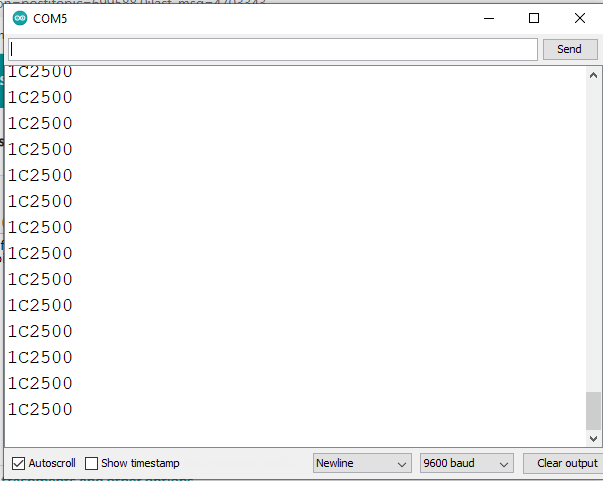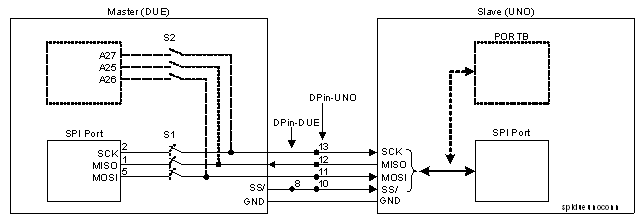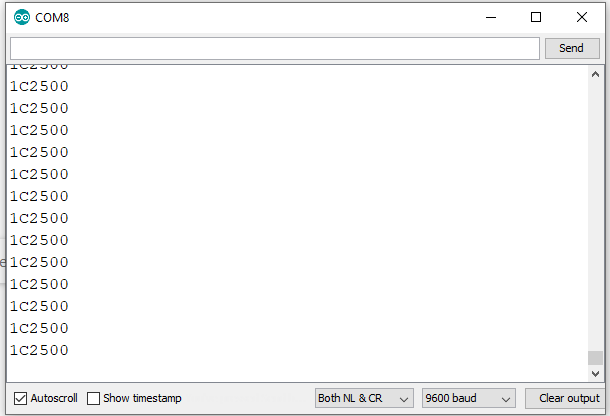# Spi communication between Arduino Due and Uno

Hello everyone. I am tired asking and since few weeks there was no reply. Can Anyone spend your valuable time looking into this.

I need to transfer 24-bit data of about 79-registers using spi protocol. I have configured Arduino Due as Master and Uno as slave. Instead of actual slave device(LMX2595) I am making use of uno. I would like to monitor the data whch is transmitted n the serial monitor window at slave side. But Unfortunately I don't get the data in sequence. Below are the code attached for Master and Slave

Master

#include <SPI.h>

SPISettings mySettting(4000000, MSBFIRST, SPI_MODE0);
const uint8_t ss = 10;

void setup() {
pinMode(ss, OUTPUT);

SPI.begin();
SPI.beginTransaction(mySettting);

digitalWrite(ss, LOW);
SPI.transfer(0x1C);
SPI.transfer(0x25);
SPI.transfer(0x00);
delay(100);
digitalWrite(ss, HIGH);

digitalWrite(ss, LOW);
SPI.transfer(0x08);
SPI.transfer(0x08);
SPI.transfer(0x01);
delay(100);
digitalWrite(ss, HIGH);

digitalWrite(ss, LOW);
SPI.transfer(0x00);
SPI.transfer(0x05);
SPI.transfer(0x02);
delay(100);
digitalWrite(ss, HIGH);

digitalWrite(ss, LOW);
SPI.transfer(0x42);
SPI.transfer(0x06);
SPI.transfer(0x03);
delay(100);
digitalWrite(ss, HIGH);

digitalWrite(ss, LOW);
SPI.transfer(0x43);
SPI.transfer(0x0A);
SPI.transfer(0x04);
delay(100);
digitalWrite(ss, HIGH);

SPI.endTransaction();
}

void loop() {
}

Slave

#include<SPI.h>

ISR (SPI_STC_vect) //Inerrrput routine function
{
}

void setup()
{
Serial.begin(4000000);

pinMode(MISO,OUTPUT); //Sets MISO as OUTPUT
SPCR |= _BV(SPE); //Turn on SPI in Slave Mode
SPI.attachInterrupt(); //Activate SPI Interuupt
}

void loop()
{
}
}

Any help will be greatly appreciated. Thanks in Advance

Is you problem due to the two processors having different logic levels?

Please remember to use code tags when posting code.

(What has this got to do with the Covid emergency?)

Thanks for the reply. I don't think so it is due to logic levels. I accept that Arduino Due operates at 3.3V where as Uno at 5V. Initially I have tried transferring string using spi protocol between Due and Uno and everything went fine. But I don't understand whats the problem with sending this 24-bit data

And the relevance to the Covid emergency is...?

Unfortunately I don't get the data in sequence.

Maybe you're not printing it fast enough

@vamshi97

Was NOT COVID related GRRR !

Your topic was Moved to it's current location / section as it is more suitable.

Please do NOT cross post / duplicate as it wastes peoples time and efforts to have more than one post for a single topic.

Continued cross posting could result in a time out from the forum.

Could you also take a few moments to Learn How To Use The Forum.
Other general help and troubleshooting advice can be found here.

As no one is replying to my query so, I don't know where to post. Could anyone solve my issue with respect to the above code posted

Are you sending the following comma separated hex-based data stream from SPI-DUE- Master to SPI-UNO-Slave?
1C2500,080801,000520,420603,430A04

And then the UNO will receive the data stream and then the data will be correctly displayed on the OutputBox of the Serial Monitor of UNO?

You can check if the examples of the attached file are helpful for you.

Ch-7OnlineLec.pdf (291 KB)

yes what you said is exactly I am trying to replicate. Thank you for the attachement.

In the meantime you try first using UNO and NANO and then try using DUE and NANO/UNO. Follow example of Section-7.4.Q3 of the pdf file attached in Post#6 to see how the Slave receives 2-byte data from Master and then expand it it to 3-byte (24-bit) and then expand it into multiple of 3-byte.

Yes I have tried the same example with Arduino Due as master and Uno as slave. But unfortunately there was no output in the serial monitor window at the slave device.

Are those referred sketches working with SPI-UNO-Master and SPI-NANO-Slave?

vamshi97:
As no one is replying to my query so, I don't know where to post. Could anyone solve my issue with respect to the above code posted

If you post your code as described in the Read this before posting a programming question, more people will read it.

The following Serial Monitor (Fig-1) of the Slave of SPI-UNO-NANO setup shows that the Slave is correctly receiving 24-bit data from Master.Figure-1:

SPI-Master-UNO Sketch:

``````#include<SPI.h>
//long x = 0x1C2500;
byte myData[] = {0x1C, 0x25, 0x00};//, 0x00};

void setup()
{
Serial.begin(9600);
SPI.begin();
SPI.setClockDivider(SPI_CLOCK_DIV16);//1 MBits/s
digitalWrite(SS, LOW);  //Slave is selected
//--------------------
}

void loop()
{
for (int i = 0; i < 3; i++)
{
SPI.transfer(myData[i]);
delayMicroseconds(100); //allows Slave to process received byte
//Serial.println(myData[i], HEX);  //shows: 0xAB
}
// Serial.println("======================");
delay(1000);  //test interval
}
``````

SPI-Slave-NANO Sketch:

``````#include<SPI.h> //must be included to get the meanings of SS, MOSI, MISO, SCK names
int i = 0;
volatile bool flag = false;

volatile byte myData;

void setup()
{
Serial.begin(9600);
SPI.setClockDivider(SPI_CLOCK_DIV16);//1 MHz speed insted of default 4 MHz
pinMode(SS, INPUT_PULLUP);  // ensure SS stays high for now
pinMode(MISO, OUTPUT);       //always do
pinMode(MOSI, INPUT);        //always do
pinMode(SCK, INPUT);         //always do
SPCR |= _BV(SPE);     //SPI logic is enabled
SPCR |= !(_BV(MSTR)); //Arduino is Slave
SPI.attachInterrupt();   //SPI interrupt logic is enabled
}

void loop()
{
if(flag == true)
{
long x = (long)myData<<16|(long)myData<<8|(long)myData;
Serial.println(x, HEX);
flag = false;
}
}

ISR(SPI_STC_vect)
{
myData[i] = SPDR;
i++;
if (i == 3)     //8-byte data are sent
{
flag = true;
i = 0;          //array pointer is reset
}
}
``````It is also working for SPI-Master-DUE and SPI-Slave-UNO. However, is it safe to operate 3.3V DUE with 5V UNO?Figure-1: Setup between DUE and UNO

1. Master Sketch

``````#include<SPI.h>
//long x = 0x1C2500;
byte myData[] = {0x1C, 0x25, 0x00};//, 0x00};

void setup()
{
Serial.begin(9600);
SPI.begin();
SPI.setClockDivider(SPI_CLOCK_DIV16);//1 MBits/s
pinMode(8, OUTPUT);
digitalWrite(8, HIGH);
delay(100);
digitalWrite(8, LOW);  //Slave is selected
//--------------------
}

void loop()
{
for (int i = 0; i < 3; i++)
{
SPI.transfer(myData[i]);
delayMicroseconds(100); //allows Slave to process received byte
//Serial.println(myData[i], HEX);  //shows: 0xAB
}
// Serial.println("======================");
delay(1000);  //test interval
}
``````

2. Slave Sketch

``````#include<SPI.h> //must be included to get the meanings of SS, MOSI, MISO, SCK names
int i = 0;
volatile bool flag = false;

volatile byte myData;

void setup()
{
Serial.begin(9600);
SPI.setClockDivider(SPI_CLOCK_DIV16);//1 MHz speed insted of default 4 MHz
pinMode(SS, INPUT_PULLUP);  // ensure SS stays high for now
pinMode(MISO, OUTPUT);       //always do
pinMode(MOSI, INPUT);        //always do
pinMode(SCK, INPUT);         //always do
SPCR |= _BV(SPE);     //SPI logic is enabled
SPCR |= !(_BV(MSTR)); //Arduino is Slave
SPI.attachInterrupt();   //SPI interrupt logic is enabled
}

void loop()
{
if (flag == true)
{
long x = (long)myData << 16 | (long)myData << 8 | (long)myData;
Serial.println(x, HEX);
flag = false;
}
}

ISR(SPI_STC_vect)
{
myData[i] = SPDR;
i++;
if (i == 3)     //8-byte data are sent
{
flag = true;
i = 0;          //array pointer is reset
}
}
``````

3. Slave's Serial Monitor (Fig-2)Figure-2:Thank you. The communication worked fine But once I repeat for more set of data I mean once I go for multiple data transfer there is an issue of repeating the data but not in a sequence.

Below is the attached code for Master and Slave.

``````[Master/]

#include<SPI.h>
byte myData[] = {0x1C, 0x25, 0x00, 0x24, 0x56, 0xA3, 0x35, 0x67, 0x98};//, 0x00};

void setup()
{
Serial.begin(9600);
SPI.begin();
SPI.setClockDivider(SPI_CLOCK_DIV16);//1 MBits/s
pinMode(8, OUTPUT);
digitalWrite(8, HIGH);
delay(100);
digitalWrite(8, LOW);  //Slave is selected
//--------------------
}

void loop()
{
for (int i = 0; i < 9; i++)
{
SPI.transfer(myData[i]);
delayMicroseconds(100);

}
delay(1000);  //test interval
}

[code][Slave]

#include<SPI.h> //must be included to get the meanings of SS, MOSI, MISO, SCK names
int i = 0;
volatile bool flag = false;

volatile byte myData;

void setup()
{
Serial.begin(9600);
SPI.setClockDivider(SPI_CLOCK_DIV16);//1 MHz speed insted of default 4 MHz
pinMode(SS, INPUT_PULLUP);  // ensure SS stays high for now
pinMode(MISO, OUTPUT);       //always do
pinMode(MOSI, INPUT);        //always do
pinMode(SCK, INPUT);         //always do
SPCR |= _BV(SPE);     //SPI logic is enabled
SPCR |= !(_BV(MSTR)); //Arduino is Slave
SPI.attachInterrupt();   //SPI interrupt logic is enabled
}

void loop()
{
if (flag == true)
{
for(i=0;i<7;i=i+3)
{
long x = (long)myData[i] << 16 | (long)myData[i+1] << 8 | (long)myData[i+2];
delay (3000);
Serial.println(x, HEX);
flag = false;
}

}
}

ISR(SPI_STC_vect)
{
myData[i] = SPDR;
i++;
if (i == 9)     //8-byte data are sent
{
flag = true;
i=0;
}
}

My final Aim is to transfer 79 registers,  24-bit by interfacing Arduino Due with actual Slave device LMX2595. Could Any one let me know the time calculation and clock synchronization.
``````

This is what the output I am getting in the serial monitor window at the slave endI have shown you the transfer of one data item. Now, you expand/condition the codes for the transfer of multiple data items. You should be able to do it -- try, try, and try.

That's fine. Could you please help me in understanding and calculating the delay as per the slave device timing requirements which is attached below. I am making use of Arduino Due which has maximum clock speed of 84 MHz and Slave device has maximum spi write speed of 75MHz. I hope mode 0 is to be used and what amount should the clock frequency divided to match the slave device.